# Raspberry Pi_Eng_25.4.1 Short and Resistance

#### Published Book on Amazon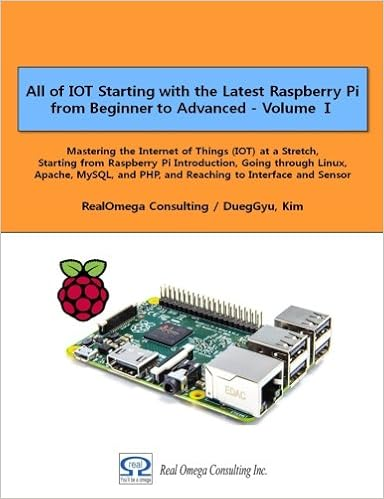All of IOT Starting with the Latest Raspberry Pi from Beginner to Advanced – Volume 1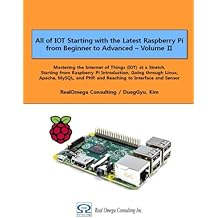All of IOT Starting with the Latest Raspberry Pi from Beginner to Advanced – Volume 2

#### 출판된 한글판 도서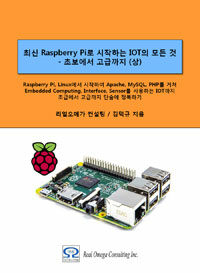최신 라즈베리파이(Raspberry Pi)로 시작하는 사물인터넷(IOT)의 모든 것 – 초보에서 고급까지 (상)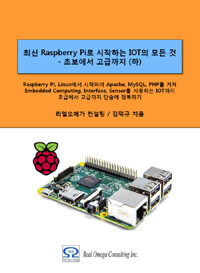최신 라즈베리파이(Raspberry Pi)로 시작하는 사물인터넷(IOT)의 모든 것 – 초보에서 고급까지 (하)

## 25.4Basics of Electronic Circuit

Here, we will explain the basic knowledge of electronic circuit.

### 25.4.1Short and Resistance

Short is also generally referred to as a short circuit, which means that two wires with different potentials are directly connected to each other, such as connecting a (+) wire and a (-) wire without a resistor in a circuit where electricity is supplied. When this direct connection is made, excessive current flows for a moment and spark occurs.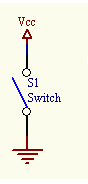The current flowing in the circuit is calculated as I = V / R. Then, if a circuit is connected to a power source without using a separate resistor, there is only a specific resistance of the wire. In this case, if a voltage of 3V ~ 5V is connected in series, theoretically the current close to infinity will flow to the wire.

For example, assuming a voltage of 3V and a resistance of 0.001Ω, a large current of I = 3 / 0.001 = 3000A flows. If you use a small power source such as a battery, the battery will not be able to supply such current, so it will not cause a big problem. However, if you connect a power source that can supply high current, heat may be generated, resulting in electric wire burning or fire.

One way to prevent the circuit from this danger is to use a resistor of the appropriate size. The reason why the overcurrent flows is that the resistance is too low. Therefore, when a resistor of an appropriate size suitable for the voltage is used, the overcurrent does not occur originally. Another way to protect the circuit from overcurrent is to attach a fuse or circuit breaker to the circuit. The fuze is that if the current exceeds a certain level, the fuze dissolves and breaks, so that the power is cut off.